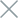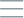• Home
• Tags
• Series
•# Evolutionary Search Algorithm in C++ - Explanation, Example, and Code

The Evolutionary Search algorithm is an optimization method based on the mechanism of natural evolution. This algorithm simulates the evolution process of individuals within a population across generations to find the best solution for a problem.

## How It Works

1. Population Initialization: Create an initial population of randomly generated individuals.
2. Evaluation: Evaluate the quality of each individual in the population based on the objective function or evaluation criteria.
3. Selection: Select a subset of the best individuals from the current population based on probabilities or selection criteria.
4. Evolution: Create a new generation by applying crossover and mutation operations to the selected individuals.
5. Iteration: Repeat steps 2 to 4 over multiple generations until a satisfactory solution is achieved or a predefined number of iterations is reached.

## Example: Optimizing the Fibonacci Function using Evolutionary Search

Consider the optimization problem of the Fibonacci function F(x) = F(x-1) + F(x-2) with F(0) = 0, F(1) = 1. We want to find the value of x for which F(x) is maximized. The Evolutionary Search method can generate a population of random x values, evolve them across generations to find the optimal x value.

## Code Example in C++

``````#include <iostream>
#include <vector>
#include <cstdlib>
#include <ctime>

int fibonacci(int n) {
if (n <= 0) return 0;
if (n == 1) return 1;
return fibonacci(n - 1) + fibonacci(n - 2);
}

int evolutionSearchFibonacci(int populationSize, int numGenerations) {
srand(time(0));

std::vector<int> population(populationSize);
for (int i = 0; i < populationSize; ++i) {
population[i] = rand() % populationSize;
}

for (int gen = 0; gen < numGenerations; ++gen) {
int bestIndex = 0;
for (int i = 1; i < populationSize; ++i) {
if (fibonacci(population[i]) > fibonacci(population[bestIndex])) {
bestIndex = i;
}
}

// Crossover and mutation operations can be applied here

// Example: Replace the worst individual with the best individual
population = population[bestIndex];
}

return population;
}

int main() {
int populationSize = 50;
int numGenerations = 100;
int result = evolutionSearchFibonacci(populationSize, numGenerations);

std::cout << "Optimal x for maximum Fibonacci value: " << result << std::endl;

return 0;
}
``````

In this example, we use the Evolutionary Search method to optimize the Fibonacci function. We generate a population of random x values, evolve them across generations by selecting the best individuals and applying crossover and mutation operations.